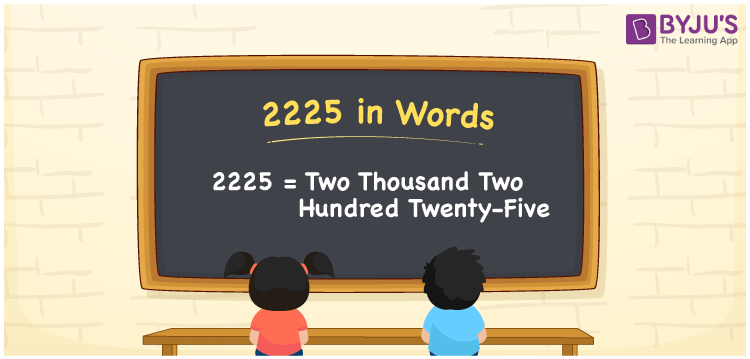# 2225 in Words

2225 in words can be written as Two Thousand Two Hundred Twenty-Five. If the daily wage of a worker is Rs. 2225, then you can say that “The daily wage of a worker is Two Thousand Two Hundred Twenty-Five Rupees”. The numbers in words topic provides complete information required to ace well in your exams. Therefore, 2225 can be read as “Two Thousand Two Hundred Twenty-Five” in English.

 2225 in words Two Thousand Two Hundred Twenty-Five Two Thousand Two Hundred Twenty-Five in Numbers 2225

## 2225 in English Words## How to Write 2225 in Words?

Let us prepare a place value chart for the four digits present in the number 2225. Using the place value of each digit, students will be able to convert the numbers into words.

 Thousands Hundreds Tens Ones 2 2 2 5

2225 in expanded form is:

2 x Thousand + 2 × Hundred + 2 × Ten + 5 × One

= 2 x 1000 + 2 × 100 + 2 × 10 + 5 × 1

= 2000 + 200 + 20 + 5

= 2225

= Two Thousand Two Hundred Twenty-Five

Therefore, 2225 in words is written as Two Thousand Two Hundred Twenty-Five.

2225 is a natural number that precedes 2226 and succeeds 2224.

2225 in words – Two Thousand Two Hundred Twenty-Five

Is 2225 an odd number? – Yes

Is 2225 an even number? – No

Is 2225 a perfect square number? – No

Is 2225 a perfect cube number? – No

Is 2225 a prime number? – No

Is 2225 a composite number? – Yes

## Frequently Asked Questions on 2225 in Words

Q1

### Write 2225 in words.

2225 can be written in words as “Two Thousand Two Hundred Twenty-Five”.
Q2

### How do you write Two Thousand Two Hundred Twenty-Five in numbers?

Two Thousand Two Hundred Twenty-Five can be written in numbers as 2225.
Q3

### Is 2225 an even number?

No, 2225 is not an even number as it is not completely divisible by 2.